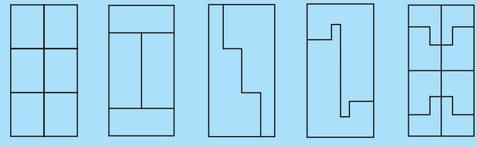# Q. 1.    Each of the following rectangles of length 6 cm and breadth 4 cm is composed of congruent polygons. Find the area of each             polygon.The total area of rectangle

i) The first rectangle is divided into 6 equal parts. So the area of each part will be one-sixth of the total area

ii) The rectangle is divided into 4 equal parts, area of each part= one-forth area of rectangle= 6 cm2

iii) and (iv) are divided into two equal parts. Area of each part will be one half the total area of rectangle= 12  cm2

v) Area of rectangle is divided into 8 equal parts. Area of one part is one-eighth of the total area of rectangle = 3 cm2

## Related Chapters

### Preparation Products

##### Knockout MET 2021

An exhaustive E-learning program for the complete preparation of MET online exam..

₹ 4999/- ₹ 2999/-
##### Knockout MET JEE Main 2021

An exhaustive E-learning program for the complete preparation of MET & JEE.

₹ 27990/- ₹ 16999/-
##### Knockout NEET May 2021

An exhaustive E-learning program for the complete preparation of NEET..

₹ 22999/- ₹ 14999/-
##### Knockout BITSAT 2020

It is an exhaustive preparation module made exclusively for cracking BITSAT..

₹ 4999/- ₹ 1999/-